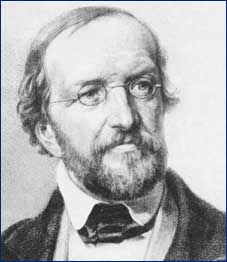A# Dirichlet, Peter Gustav Lejeune (1805–1859)Peter Dirichlet was a German mathematician who made significant contributions to number theory, analysis, and mechanics, and who is credited with the modern formal definition of a function. He taught at the universities of Breslau (1827) and Berlin (1828–1855) and in 1855 succeeded Carl Gauss at the University of Göttingen but died of a heart attack only three years later. Dirichlet continued Gauss's great work on number theory, publishing on Diophantine equations of the form x 5 + y 5 = kz 5. His book Lectures on Number Theory (1863) is similar in stature to Gauss's earlier Disquisitiones and founded modern algebraic number theory. In 1829 he gave the conditions sufficient for a Fourier series to converge (though the conditions necessary for it to converge are still undiscovered).

## Dirichlet's theorem

Dirichlet's theorem states that for any two positive coprime integers, a and b, there are infinitely many prime numbers of the form a n + b, where n > 0. This theorem was first conjectured by Karl Gauss and proved by Peter Dirichlet in 1835.